How to Split Numbers and Text from String in Excel

Many times I get mixed data from field and server for analysis. This data is usually dirty, having column mixed with number ans text. While doing data cleaning before analysis, I separate numbers and text in separate columns. In this article, I’ll tell you how you can do it.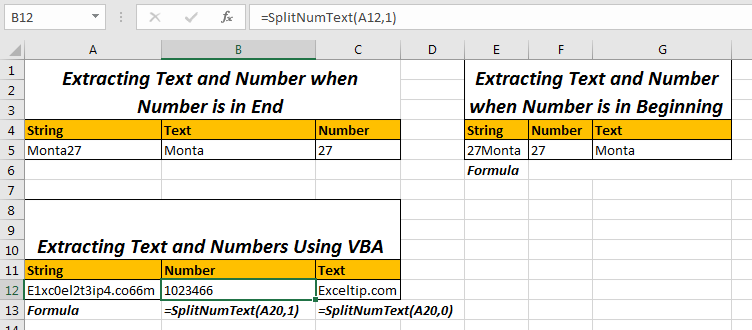Scenario:
So one our friend on Exceltip.com asked this question in the comments section. “How do I separate numbers coming before a text and in the end of text using excel Formula. For example 125EvenueStreet and LoveYou3000 etc.”

To extracting text, we use RIGHT, LEFT, MID and other text functions. We just need to know the number of texts to extract. And Here we will do the same first.
Extract Number and Text from a String when Number is in End of String
For above example I have prepared this sheet. In Cell A2, I have the string. In cell B2, I want the text part and in C2 the Number Part.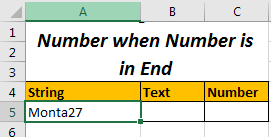So we just need to know the position from where number starts. Then we will use Left and other function. So to get position of first number we use below generic formula:
Generic Formula to Get Position of First Number in String:

=MIN(SEARCH({0,1,2,3,4,5,6,7,8,9},String_Ref&"0123456789")

This will return the position of first number.
For above example write this formula in any cell.

=MIN(SEARCH({0,1,2,3,4,5,6,7,8,9},A5&"0123456789"))

Extract Text Part

It will return 15 as the first number which is found is at 15th position in Text. I will explain it later.

Now, to get Text, from left we just need to get 15-1 character from string. So we will use
LEFT function to extract text.
Formula to Extract Text from Left

=LEFT(A5,MIN(SEARCH({0,1,2,3,4,5,6,7,8,9},A5&"0123456789"))-1)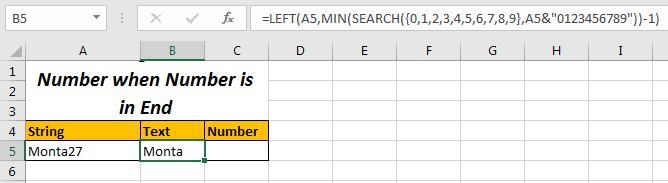Here we just subtracted 1 from what ever number returned by MIN(SEARCH({0,1,2,3,4,5,6,7,8,9},A5&"0123456789")).
Extract Number Part

Now to get numbers we just need to get number characters from 1st number found. So we calculate the total length of string and subtract the position of first number found and add 1 to it. Simple. Yeah it’s just sound complex, it is simple.
Formula to Extract Numbers from Right

=RIGHT(A5,LEN(A5)-MIN(SEARCH({0,1,2,3,4,5,6,7,8,9},A5&"0123456789"))+1)

Here we just got total length of string using LEN function and then subtracted the position of first found number and then added 1 to it. This gives us total number of numbers. Learn more here about extracting text using LEFT and RIGHT functions of Excel.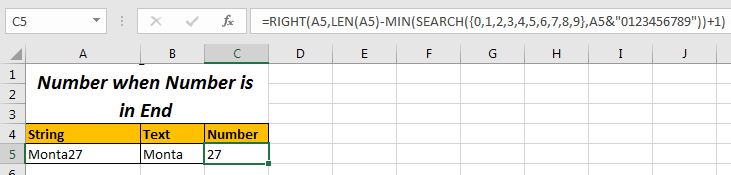So the LEFT and RIGHT function part is simple. The Tricky part is MIN and SEARCH Part that gives us the position of first found number. Let’s understand that.
How It Works
We know how LEFT and RIGHT function work. We will explore the main part of this formula that gets the position of first number found and that is: MIN(SEARCH({0,1,2,3,4,5,6,7,8,9},String&"0123456789")
The SEARCH function returns the position of a text in string. SEARCH(‘text’,’string’) function takes two argument, first the text you want to search, second the string in which you want to search.

• Here in SEARCH, at text position we have an array of numbers from 0 to 9. And at string position we have string which is concatenated with "0123456789" using & operator. Why? I’ll tell you.
• Each element in the array {0,1,2,3,4,5,6,7,8,9} will be searched in given string and will return its position in array form string at same index in array.
• If any value is not found, it will cause an error. Hence all formula will result into an error. To avoid this, we concatenated the numbers "0123456789" in text. So that it always finds each number in string. These numbers are in the end hence will not cause any problem.
• Now The MIN function returns the smallest value from array returned by SEARCH function. This smallest value will be the first number in string. Now using this NUMBER and LEFT and RIGHT function, we can split the text and string parts.

Let’s examin our example. In A5 we have the string that has street name and house number. We need to separate them in different cells.
First let’s see how we got our position of first number in string.

• MIN(SEARCH({0,1,2,3,4,5,6,7,8,9},A5&"0123456789")): this will translate into MIN(SEARCH({0,1,2,3,4,5,6,7,8,9},”Monta270123456789”))

Now, as I explained search will search each number in array {0,1,2,3,4,5,6,7,8,9} in Monta270123456789 and will return its position in an array form. The returned array will be {8,9,6,11,12,13,14,7,16,17}. How?
0 will be searched in string. It is found at 8 position. Hence our first element is 8. Note that our original text is only 7 characters long. Get it. 0 is not a part of Monta27.
Next 1 will be searched in string and it is also not part of original string, and we get it’s position 9.
Next 2 will be searched. Since it is the part of original string, we get its index as 6.
Similarly each element is found at some position.

• Now this array is passed to MIN function as MIN({8,9,6,11,12,13,14,7,16,17}). MIN returns the 6 which is position of first number found in original text.
And the story after this is quite simple. We use this number extract text and numbers using LEFT and RIGHT Function.

Extract Number and Text from a String When Number is in Beginning of String
In Above example, Number was in the end of the string. How do we extract number and text when number is in the beginning.

I have prepared a similar table as above. It just has number in the beginning.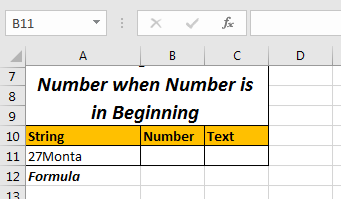Here we will use a different technique. We will count the length of numbers (which is 2 here) and will extract that number of characters from left of String.
So the method is =LEFT (string, count of numbers)
To Count the Number of characters this is the Formula.
Generic Formula to Count Number of Numbers:

=SUM(LEN(string)-LEN(SUBSTITUTE(string,{"0","1","2","3","4","5","6","7","8","9"},""))

Here,

• SUBSTITUTE function will replace each number found with “” (blank). If a number is found ti substituted and new string will be added to array, other wise original string will be added to array. In this way, we will have array of 10 strings.
• Now LEN function will return length of characters in an array of those strings.
• Then, From length of original strings, we will subtract length of each string returned by SUBSTITUTE function. This will again return an array.
• Now SUM will add all these numbers. This is the count of numbers in string.

Extract Number Part from String

Now since we know the length of numbers in string, we will substitute this function in LEFT.
Since We have our string an A11 our:

Formula to Extract Numbers from LEFT

=LEFT(A11,SUM(LEN(A11)-LEN(SUBSTITUTE(A11,{"0","1","2","3","4","5","6","7","8","9"},""))))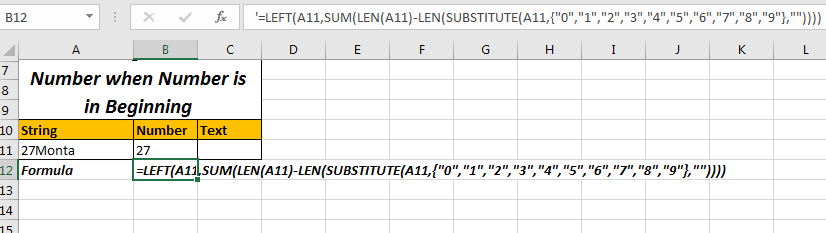Extract Text Part from String

Since we know number of numbers, we can subtract it from total length of string to get number alphabets in string and then use right function to extract that number of characters from right of the string.

Formula to Extract Text from RIGHT

=RIGHT(A11,LEN(A2)-SUM(LEN(A11)-LEN(SUBSTITUTE(A11,{"0","1","2","3","4","5","6","7","8","9"},""))))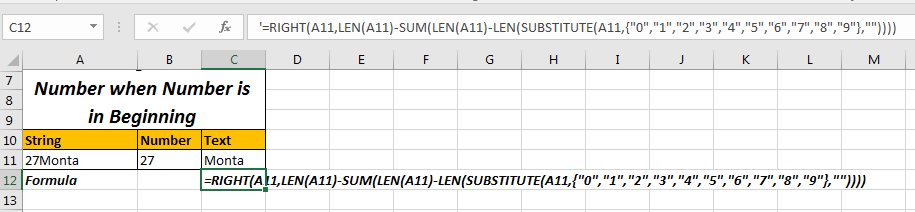How it Works
The main part in both formula is SUM(LEN(A11)-LEN(SUBSTITUTE(A11,{"0","1","2","3","4","5","6","7","8","9"},""))) that calculates the first occurance of a number. Only after finding this, we are able to split text and number using LEFT function. So let’s understand this.

• SUBSTITUTE(A11,{"0","1","2","3","4","5","6","7","8","9"},""): This part returns an array of string in A11 after substituting these numbers with nothing/blank (“”). For 27Monta it will return {"27Monta","27Monta","7Monta","27Monta","27Monta","27Monta","27Monta","2Monta","27Monta","27Monta"}.
• LEN(SUBSTITUTE(A11,{"0","1","2","3","4","5","6","7","8","9"},"")): Now the SUBSTITUTE part is wrapped by LEN function. This return length of texts in array returned by SUBSTITUTE function. In result, we’ll have {7,7,6,7,7,7,7,6,7,7}.
• LEN(A11)-LEN(SUBSTITUTE(A11,{"0","1","2","3","4","5","6","7","8","9"},"")): Here we are subtracting each number returned by above part from length of actual string. Length of original text is 7. Hence we will have {7-7,7-7,7-6,....}. Finally we will have {0,0,1,0,0,0,0,1,0,0}.
• SUM(LEN(A11)-LEN(SUBSTITUTE(A11,{"0","1","2","3","4","5","6","7","8","9"},""))): Here we used SUM to sum the array returned by above part of function. This will give 2. Which is number of numbers in string.

Now using this we can extract the texts and number and split them in different cells. This method will work with both type text, when number is in beginning and when its in end. You just need to utilize LEFT and RIGHT Function appropriately.
Use SplitNumText function To Split Numbers and Texts from A String
The above to methods are little bit complex and they are not useful when text and numbers are mixed. To split text and numbers use this user defined function.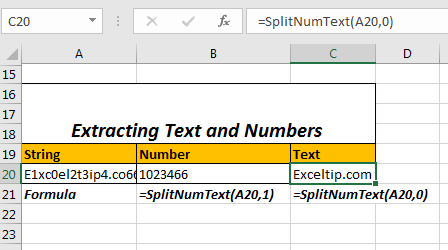Syntax:

=SplitNumText(string, op)

String: The String You want to split.
Op: this is boolean. Pass 0 or false to get text part. For number part, pass true or any number greater then 0.
For example, if the string is in A20 then,
Formula for extracting numbers from string is:

=SplitNumText(A20,1)

And
Formula for extracting text from string is:

=SplitNumText(A20,0)

Copy below code in VBA module to make above formula work.

Function SplitNumText(str As String, op As Boolean)
num = ""
txt = ""
For i = 1 To Len(str)
If IsNumeric(Mid(str, i, 1)) Then
num = num & Mid(str, i, 1)
Else
txt = txt & Mid(str, i, 1)
End If
Next i
If op = True Then
SplitNumText = num
Else
SplitNumText = txt
End If
End Function

This code simply checks each character in string, if its a number or not. If it is a number then it is stored in num variable else in txt variable. If user passes true for op then num is returned else txt is returned.

This is the best way to split number and text from a string in my opinion.

So yeah guys, these are the ways to split text and numbers in different cells. Let me know if you have any doubts or any better solution in the comments section below. Its always fun to interact with guys.

1.Hi,

I want to ask how I can separate /extract the numbers before the (-) sign,

123456-8754689
1212-8900876

I'm having a hard time because the number of digits before the hyphen is not the same.

Mai from Japan.

2.I have another case like under cell value.

15Artsign/Multidesk Stand/F9025/100*90*22/A7 (A1)

I want separate like under.

15 (B1)

Artsign/Multidesk Stand/F9025/100*90*22/A7 (C1)

Sincerely yours,

Lee Joo Hyun form South Korea.

•Hi Lee,

This can be easily done by the formula in B1
=Left(A1,2)
then in C1 write below formula
=RIGHT(A1,LEN(A1)-LEN(B1))

Terms and Conditions of use

The applications/code on this site are distributed as is and without warranties or liability. In no event shall the owner of the copyrights, or the authors of the applications/code be liable for any loss of profit, any problems or any damage resulting from the use or evaluation of the applications/code.